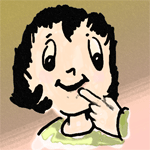#### You may also like### Pebbles

Place four pebbles on the sand in the form of a square. Keep adding as few pebbles as necessary to double the area. How many extra pebbles are added each time?### Bracelets

Investigate the different shaped bracelets you could make from 18 different spherical beads. How do they compare if you use 24 beads?### Sweets in a Box

How many different shaped boxes can you design for 36 sweets in one layer? Can you arrange the sweets so that no sweets of the same colour are next to each other in any direction?

# Picture a Pyramid ...

##### Age 7 to 11Challenge LevelYou will probably have to write some numbers down.
BUT
Try not to draw anything.
Here we go ...

Imagine a step pyramid.
Each layer is made from a number of stone cubes.
There is a square number of cubes in each layer, which increases for each layer (increasing from top to bottom).
The cubes are numbered starting from the top layer so that, looking from above, each new layer carries on counting starting from the North West corner. (When the end of a row is reached you start at the West end of the next row.)
There are equal steps on all four sides of the pyramid (in other words, each successive layer "sits" exactly in the middle of the layer beneath it).

We'll imagine a six-layered pyramid that uses 91 cubes in total.What numbered cubes are vertically underneath (in a straight line):

the cube that is number 1?

the cube that is number 4?

the cube that is number 8?

Now imagine stepping up two layers at a time, starting at the middle cube on the South side, second layer up. Which numbered cubes would you step on to end at the top, if you stepped up in a straight line?

Starting at the South West Corner and stepping up one layer at a time on the corner steps, which numbered cubes would you step on?

What if a second pyramid was built, numbering the cubes as they built the pyramid, so the numbering would start from the bottom North West corner and starting the second row as before.
So number 1 at the bottom and the last cube number 91.

What numbered cubes are vertically underneath (in a straight line):

the cube that is number 91?

the cube that is number 89?

the cube that is number 80?

Is there a cube that has the same number in both pyramids?

What was the difference for you in doing the second pyramid compared with doing the first pyramid?